# 逻辑回归

### Sigmoid函数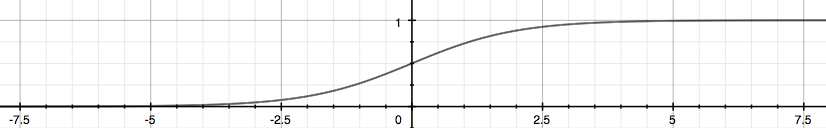### Linear Decision Boundary

• $\theta^{(T)}x ≥ 0$ 可推出 $y=1$
• $\theta^{(T)}x < 0$ 可推出 $y=0$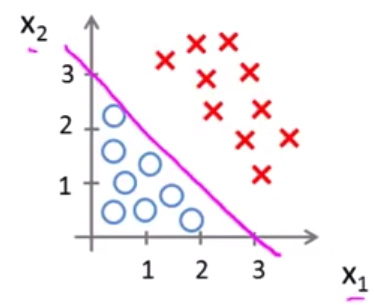### Non-linear Decision Boundary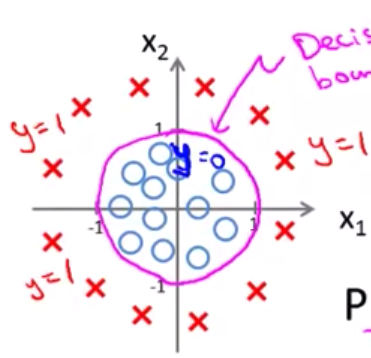### Cost Function

y=1的时候，J(θ) = 0 -> h(x)=1J(θ) = ∞ -> h(x)=0，如下图所示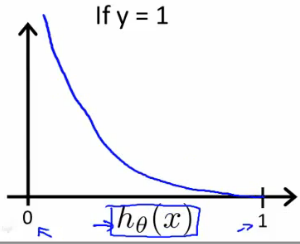y=0的时候，J(θ) =0 -> h(x)=0J(θ) = ∞ -> h(x)=1，如下图所示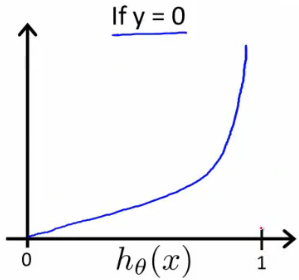### Simplifed Cost Function

$\begin{array}{rl}& Repeat\phantom{\rule{thickmathspace}{0ex}}\left\{\\ & \phantom{\rule{thickmathspace}{0ex}}{\theta }_{j}:={\theta }_{j}-\alpha \frac{\mathrm{\partial }}{\mathrm{\partial }{\theta }_{j}}J\left(\theta \right)\\ & \right\}\end{array}$

${J}_{\left(\theta \right)}$求偏导，得到梯度下降公式：

$\begin{array}{rl}& Repeat\phantom{\rule{thickmathspace}{0ex}}\left\{\\ & \phantom{\rule{thickmathspace}{0ex}}{\theta }_{j}:={\theta }_{j}-\frac{\alpha }{m}\sum _{i=1}^{m}\left({h}_{\theta }\left({x}^{\left(i\right)}\right)-{y}^{\left(i\right)}\right){x}_{j}^{\left(i\right)}\\ & \right\}\end{array}$

$\theta :=\theta -\frac{\alpha }{m}{X}^{T}\left(g\left(X\theta \right)-\stackrel{\to }{y}\right)$

• BFGS
• L-BFGS

• 不需要人工选择α
• 比低度下降更快

$\begin{array}{rl}& J\left(\theta \right)\\ & \frac{\mathrm{\partial }}{\mathrm{\partial }{\theta }_{j}}J\left(\theta \right)\end{array}$

function [jVal, gradient] = costFunction(theta)
jVal = [...code to compute J(theta)...];
gradient = [...code to compute derivative of J(theta)...];
end


options = optimset('GradObj', 'on', 'MaxIter', 100);
initialTheta = zeros(2,1);
[optTheta, functionVal, exitFlag] = fminunc(@costFunction, initialTheta, options);


fminunc()函数接受三个参数：costFunction，初始的 θ 值（至少是 2x1 的向量），还有 options

### Multiclass classification

• Email foldering/tagging：Work，Friends，Family，Hobby
• Weather：Sunny，Cloudy，Rain，Snow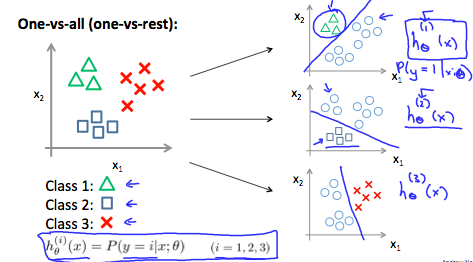$\begin{array}{rl}& y\in \left\{0,1...n\right\}\\ & {h}_{\theta }^{\left(0\right)}\left(x\right)=P\left(y=0|x;\theta \right)\\ & {h}_{\theta }^{\left(1\right)}\left(x\right)=P\left(y=1|x;\theta \right)\\ & \cdots \\ & {h}_{\theta }^{\left(n\right)}\left(x\right)=P\left(y=n|x;\theta \right)\\ & \mathrm{p}\mathrm{r}\mathrm{e}\mathrm{d}\mathrm{i}\mathrm{c}\mathrm{t}\mathrm{i}\mathrm{o}\mathrm{n}=\underset{i}{max}\left({h}_{\theta }^{\left(i\right)}\left(x\right)\right)\end{array}$
• One-vs-all(one-vs-rest):

Train a logistic regression classifier${h}_{\theta }^{\mathrm{\left(i\right)}}\mathrm{\left(x\right)}$ for each class $i$ to predict the probability that $y=i$.

On a new input $x$, to make a prediction, pick the class $i$ that maximizes

• 总结一下就是对每种分类先计算他的${h}_{\theta }\mathrm{\left(x\right)}$，当有一个新的x需要分类时，选一个让${h}_{\theta }\mathrm{\left(x\right)}$值最大的分类器。

### The problem of overfitting• 通过线性函数预测房价不够精确(underfit)，术语叫做”High Bias”，其原因主要是样本的 feature 太少了。
• 通过二次函数拟合出来的曲线刚好可以贯穿大部分数据样本，术语叫做”Just Right”
• 通过四阶多项式拟合出来的曲线虽然能贯穿所有数据样本，但是曲线本身不够规则，当有新样本出现时不能很好的预测。这种情况我们叫做Over Fitting（过度拟合），术语叫做”High variance”。If we have too many features, the learned hypothesis may fit the training set very well（J(θ)=0）, but fail to generalize to new examples(predict prices on new examples) Over Fitting 的问题在样本少，feature 多的时候很明显

• Addressing overfitting: - Reduce number of features - Manually select which features to keep. - Model selection algorithm - Regularization - Keep all features, but reduce magnitude/values of parameters ${\theta }_{j}$ - Works well when we have a lot of features, each of which contributes a bit to predicting$y$

### Regularization Cost Function

${\theta }_{0}+{\theta }_{1}x+{\theta }_{2}{x}^{2}+{\theta }_{3}{x}^{3}+{\theta }_{4}{x}^{4}$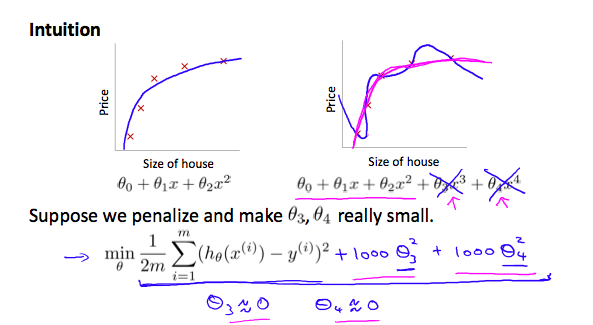Small values for parameters ${\theta }_{0},{\theta }_{1},...,{\theta }_{n}$

• “Simpler” hypothesis，选取更小的θ值能得到更简单的预测函数，例如上面的例子，如果将 ${\theta }_{3},{\theta }_{4}$近似为 0 的话，那么上述函数将变为二次方程，更贴近合理的假设函数
• Housing example: - Feature: ${x}_{0},{x}_{1},...,{\theta }_{100}$ - Parameters: ${\theta }_{0},{\theta }_{1},...,{\theta }_{100}$

100 个 feature，如何有效的选取这些θ呢，改变 cost function：

$J\left(\theta \right)=\frac{1}{2m}\left[\sum _{i=1}^{m}\left({h}_{\theta }\left({x}^{\left(i\right)}\right)-{y}^{\left(i\right)}{\right)}^{2}+\lambda \sum _{j=1}^{n}{\theta }_{j}^{2}\right]$

### Regularized linear regression

• 修改梯度下降公式为：

$\frac{\lambda }{m}{\theta }_{j}$提出来，得到:

${\theta }_{j}:={\theta }_{j}\left(1-\alpha \frac{\lambda }{m}\right)-\alpha \frac{1}{m}\sum _{i=1}^{m}\left({h}_{\theta }\left({x}^{\left(i\right)}\right)-{y}^{\left(i\right)}\right){x}_{j}^{\left(i\right)}$

• 应用到 Normal Equation

L 是一个（n+1)x(n+1)的对单位阵，第一项是 0。在引入 λ.L 之后${X}^{T}X+\lambda L$保证可逆

### Regularized logistic regression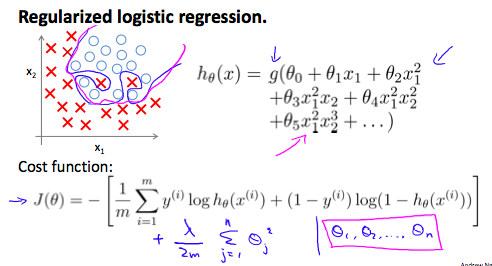• Octave Demo
function [J, grad] = lrCostFunction(theta, X, y, lambda{

%LRCOSTFUNCTION Compute cost and gradient for logistic regression with
%regularization
%   J = LRCOSTFUNCTION(theta, X, y, lambda) computes the cost of using
%   theta as the parameter for regularized logistic regression and the
%   gradient of the cost w.r.t. to the parameters.

% Initialize some useful values
m = length(y); % number of training examples

% You need to return the following variables correctly
J = 0;

h = sigmoid(X*theta); %  X:118x28, theta:28x1 h:118x1

%向量化实现
J = 1/m * (-y'*log(h)-(1-y)'*log(1-h)) + 0.5*lambda/m * sum(theta([2:end]).^2);
%代数形式实现
%J = 1/m * sum((-y).*log(h) - (1-y).*log(1-h)) + 0.5*lambda/m * sum(theta([2:end]).^2);

r = lambda/m .* theta;
r(1) = 0; %skip theta(0)